Select Page

Computer Organization

Memory Organization
Interrupt
DMA Controller

# Simplify Boolean Expressions Using K-MAP

The K-Map method is a simple and efficient method for simplify Boolean Expressions. In this lecture, we will learn to solve two and three variables Boolean functions using K-MAP. This method is also known as Karnaugh Map.

K-MAP is represented in the form of Truth Table. In a truth table, a function of n variables will have 2^n min-terms. For example, there will be four min-terms in the case of two variables i.e.(x,y) and 8 min-terms for 3 variables.

Note: Min-terms and Max-terms are forms of Literals.

## Two-Variable K-Map

The 0 and 1 are representing the row, and column. X and Y are variables of a Boolean function. The following diagram shows the arrangement of two variables Boolean function in the form of K-MAP.

• According to Pic A, Square in Row 0, and Column 0 is m0. Square in Row 0 and Column 1 is m1. M0, m1, m2… m (n) are the min-terms.
• According to Pic B, Square in Row 0, and Column 0 is X’.Y’ and so on.### Example 01: Simplify the Boolean Function F(X, Y) = x.y+x’y

Step 01: Represent the function in the form of K-MAP

Represent the corresponding boxes of (x.y) and (x’.y) with 1 and remaining with 0.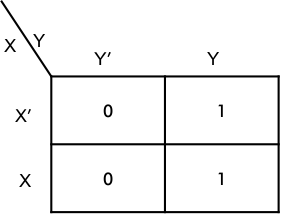Step 02: mark any group of two or for adjacent 1 as shown below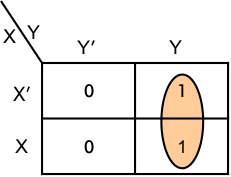Step 03: write a simplified expression for each group

X’.y+x.y = (x’+x).y = 1.y =y

Step 04: write the final simplified form as a sum of products

F(X, Y) = y

### Example 02: Simplify the Boolean Function F(X, Y) = x.y’+x’.y+x.y

Step 01: Represent the function in the form of K-MAP

Represent the corresponding boxes of (x.y’), (x’.y) and (x.y) with 1 and remaining with 0.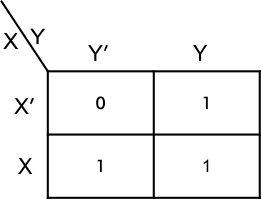Step 02: mark any group of two or for adjacent 1 as shown below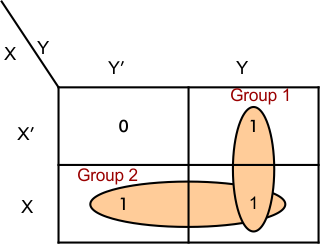Step 03: write simplified expression for each group

Group 01: X’.y+x.y = (x’+x).y = 1.y =y

Group 02: X.y’+x.y = (y’+y).x = 1.x =x

Step 04: write the final simplified form as a sum of products

F(X, Y) = x+y

### Example 03: Simplify the Boolean Function F(X, Y) = x’.y’+ x.y’+x’.y+x.y

Step 01: Represent the function in the form of K-MAP

Represent the corresponding boxes of (x’,y’),(x.y’), (x’.y) and (x.y) with 1 and remaining with 0.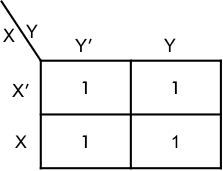Step 02: mark any group of two or for adjacent 1 as shown below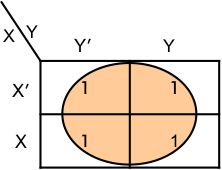Step 03: write simplified expression for each group

As all boxes are filled with one, so there will be only one group.

Group 01: x’.y’+ x.y’+x’.y+x.y = y’(x+x’)+ y(x+x’)= y’.1+y.1= y’+y =  1

Step 04: write the final simplified form as a sum of products

F(X, Y) = 1

## Three-Variable K-Maps

There are eight min-terms in a three-variable map. Therefore, the map consists of eight squares.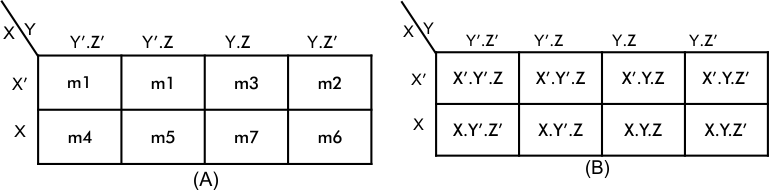let explain some examples of three-variables Boolean algebra simplifications using the K-Map method

### Example 01: Simplify the Boolean Function F(X, Y, Z) = x.y.z’+ x’.y’.z’+ x.y’.z’+x’.y.z

Step 01: Represent the function in the form of K-MAP

Represent the corresponding boxes of x.y.z’,  x’.y’.z’, x.y’.z’ and x’.y.z with 1 and remaining with 0.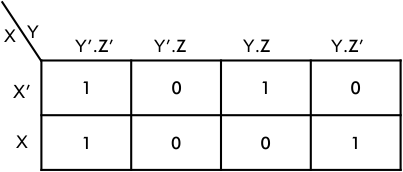Step 02: mark any group of two or for adjacent 1 as shown below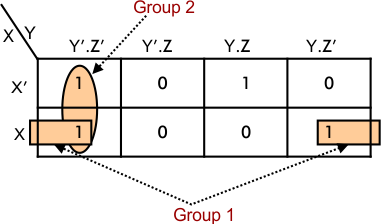Step 03: write simplified expression for each group

Group 01: x’.y’.z’ + x.y’.z’ = y’.z’(x+x’) = y’.z’(1)= y’.z’

Group 01:  x.y’.z’ + x.y.z’    = x.z’(x+x’) = x.z’(1) = x.z’

Step 04: write the final simplified form as a sum of products

F(X, Y) = y’.z’+ x.z’

Help Other’s By Sharing…